# Substract all values in a rows from the maximum value in a group

I am doing analysis of some election data, I need to calculate the losing margin of each candidate in a particular seat. Example below, can someone pls advise how I can do that?
The calculation is simple - Votes of each candidates minus the max votes of that seat

Welcome to the forum @sal.

Try using Group Loop start node to iterate through the various groups one by one. A Math Formula node will allow you to do the math. Finish with a Loop End node.

3 Likes

Hi @sal , and welcome to the Knime Community. The solution would be how @elsamuel suggested.

We can put something together if it’s not clear for you, however, it’s better if you share some data that we can use. It’s hard for us having to manually enter what you are showing us in a screenshot.

1 Like

Knime_Example.xlsx (11.4 KB)
here you go bruno, it would be great if you can put something together. Thanks!

Hi @sal

Take a look a this wf substract_from_max.knwf (26.9 KB) .
It uses a Group Loop (doing a calculation for every group of “Seat” with the Math Formula node (`COL_MAX(\$Votes\$) - \$Votes\$`)
gr, Hans

3 Likes

Here you go @sal , workflow looks like this: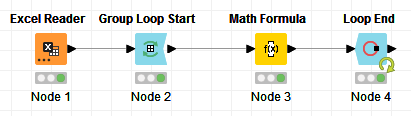I added the results in a new column called “Knime Losing Margin” so you can compare to what you got from Excel:

As you can see, the results are the same.

Here’s the workflow: Substract all values in a rows from the maximum value in a group.knwf (23.0 KB)

4 Likes

Thanks @bruno29a. Really appreciate it!

1 Like

Thanks @HansS . Really appreciate it!

1 Like

examples of whats inside each node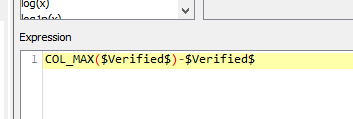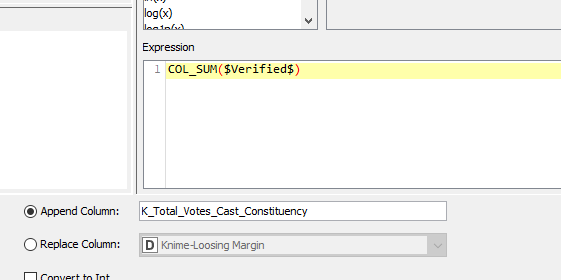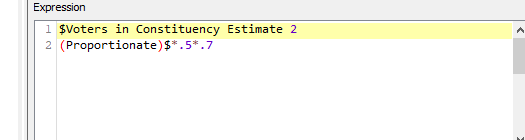Hi @sal , I put something together for your 2 example requests.

This sort of if then else logic can usually be tackled by a Rule-Engine node with:

``````\$party\$ = "X" => someValue
TRUE => anotherValue
``````

The problem here is that `someValue` and `anotherValue` cannot be calculated within the Rule Engine, that is you cannot do operations such as Votes+2 or Votes+3. You can assigned a fixed value, or point to a column.

So, this does not work. Similarly with the Math Formula node, you can do operations (math operations that is), but you can’t do the kind of if then else that we want to do (there is some if then else, but not applicable on string columns). So this does not work either.

In this case, you want to use Column Expressions: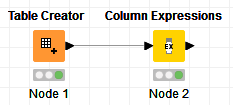Input: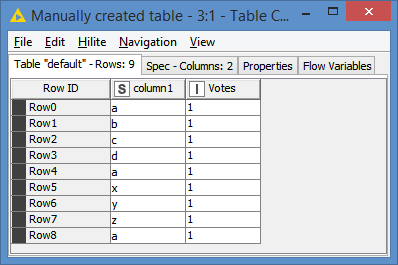And for test, I want to do if column1 is “a”, then do Votes+2, otherwise Votes+3:

Results: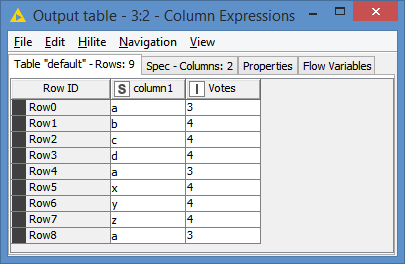Explanation:
I created an expression like this:

``````if(column("column1") == "a") {
} else {
}
``````

And I chose to replace the Votes column with the result.
So, basically the code is reading if the column column1 is “a” then I calculate value from column Votes +2, otherwise I calculate value from column Votes. I added the function `toInt()` to make sure that the results stay as an integer. On some version of Knime, the results would actually come out as a Double (float).

Since I chose to replace the column, the results of the above logic will end up in the Votes column, hereby replacing the original values with the results.

You can add as many expressions as you want in the same Column Expressions for as many different columns as you want.

I’ll do 2 examples of it: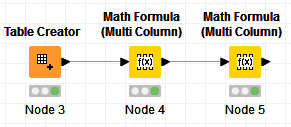Input data: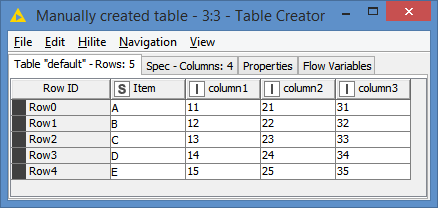The first rule to remember about the Math Formula nodes - both Math Formula or Math Formula (Multi Column), is that they can only be used with columns of type number. I purposely added a String column `Item` in the example.

When you try to configure the Math Formula nodes on this table, you will have access only to the columns of type number, and therefore not to the string column `Item`:

As you can see, only the integer columns are available for selection.

Now, about Math Formula (Multi Column), what you have to know is that the same operation will be applied to all the columns. You cannot do different operations for different columns in the same node. You can use additional Math Formula (Multi Column) nodes if you have additional operations to perform (more about this later).

In this node, I want to do an operation where I’m doing X2 on the columns column2 and column3. In this case, I want to include these 2 columns, and exclude column1:

So, the operation of X2 will be applied only to column2 and column3.

Normally, if it was the regular Math Formula node, you would do:
`\$column2\$ * 2` for column2, and
`\$column3\$ * 2` for column 3.

The Math Formula (Multi Column) main purpose is that you can do that operation for multiple columns without having to write the expression multiple times. So the expression would be written like this:
`\$\$CURRENT_COLUMN\$\$ * 2`

You can think of the node going through each of the columns that you selected (column2 and column3 in this case) one by one, and at each iteration, it would replace `\$\$CURRENT_COLUMN\$\$` by the current column of the iteration, that is, in our case, 1st iteration would be column2, and 2nd iteration would be column 3:

In this case, I chose to replace the columns with the results, so column2 and column3 will have new values (original values x 2):For the other Math Formula (Multi Column), I want to do a similar operation as what you did for the Losing Margin, and I want to do this this on all of the columns. I also want to add this result in new columns instead of overwritten the original values.
Note: Since this node goes after the previous Math Formula (Multi Column), that means that the input data for this node is what the output of the previous node was, which is the last screenshot.

So, the new node is configured as follows:

Results:

As you can see, I performed 2 different operations (x2 and the Losing Margin) on multiple columns, but each operation had to be done in separate nodes.

Here’s the workflow with both examples (Column Expressions and Math Formula multi column): Example of Column Expressions and Math Formula multi column.knwf (12.3 KB)

4 Likes

Thanks @bruno29a for the elaborate discussion

1 Like

This topic was automatically closed 7 days after the last reply. New replies are no longer allowed.